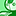# New to Qlik Sense

If you’re new to Qlik Sense, start with this Discussion Board and get up-to-speed quickly.

Announcements
Qlik Insider: SaaS capabilities for Data Integration and Analytics, May 25th! Register Now
cancel
Showing results for
Did you mean:Creator II

## Qlik Sense Set Analysis and Expression Help

Hi Qlik Experts,

I have the following requirement.

Amount 1

Sum({<Status={'Active'}>}Finance Amount)

Amount 2

Sum({<Status={'Passive'}>}Finance Amount)

I want the List of Proects where Amount 1 > Amount 2 and there corresponding Amount 1 where satisfies the condition that is Amount 1> Amount 2.

When I tried the below expression I am getting the projects where Amount 1 > Amount 2 and Amount 1 = Amount 2

Sum(Aggr(if(Amount 1> Amount 2, Amount 1), Project Number)).

Thanks

Hari

1 Solution

Accepted SolutionsCreator III

Is your problem that you only want the Amount1 where Amount1 is greater than Amount2, and not where they are equal?

Is there any chance that you are looking at two values that are being rounded to the same amount for display purposes, but underneath the hood one is actually bigger than the other? e.g.:

Amount 1: 100.01

Amount 2: 100.001

Rounded to the ones place they'll both be 100, but in expression Amount1 > Amount2

5 RepliesCreator II

Hi,

I think you should create Amount1 and Amount2 as variables

Then use Sum(Aggr(if(\$(Amount1)> \$(Amount2), \$(Amount1 )), Project Number)).

Thanks!Creator III

Is your problem that you only want the Amount1 where Amount1 is greater than Amount2, and not where they are equal?

Is there any chance that you are looking at two values that are being rounded to the same amount for display purposes, but underneath the hood one is actually bigger than the other? e.g.:

Amount 1: 100.01

Amount 2: 100.001

Rounded to the ones place they'll both be 100, but in expression Amount1 > Amount2Partner

Hi Hari,

Try like this

Sum(Aggr(if(Sum({<Status={'Active'}>}Finance Amount)>= Sum({<Status={'Passive'}>}Finance Amount), Sum({<Status={'Active'}>}Finance Amount)), Project Number))

Regards,

Jagan.Partner

One more thing you have to check is decimal points?  Are you rounding off any values?

If round off everywhere.

Regards,

Jagan.Partner

Also you have to apply set analysis for outer Sum() also.

Regards,

Jagan.